# Samacheer Kalvi Books: Tamilnadu State Board Text Books Solutions

## Samacheer Kalvi 11th Chemistry Chapter 3 Periodic Classification of Elements Notes PDF Download: Tamil Nadu STD 11th Chemistry Chapter 3 Periodic Classification of Elements NotesSamacheer Kalvi 11th Chemistry Chapter 3 Periodic Classification of Elements Notes PDF Download: Tamil Nadu STD 11th Chemistry Chapter 3 Periodic Classification of Elements Notes

## Samacheer Kalvi 11th Chemistry Chapter 3 Periodic Classification of Elements Notes PDF Download

We bring to you specially curated Samacheer Kalvi 11th Chemistry Chapter 3 Periodic Classification of Elements Notes PDF which have been prepared by our subject experts after carefully following the trend of the exam in the last few years. The notes will not only serve for revision purposes, but also will have several cuts and easy methods to go about a difficult problem.

 Board Tamilnadu Board Study Material Notes Class Samacheer Kalvi 11th Chemistry Subject 11th Chemistry Chapter Chapter 3 Periodic Classification of Elements Format PDF Provider Samacheer Kalvi Books

## How to Download Samacheer Kalvi 11th Chemistry Chapter 3 Periodic Classification of Elements Notes PDFs?

2. Click on the Samacheer Kalvi 11th Chemistry Notes PDF.
3. Look for your preferred subject.
4. Now download the Samacheer Kalvi 11th Chemistry Chapter 3 Periodic Classification of Elements notes PDF.

## Download Samacheer Kalvi 11th Chemistry Chapter 3 Periodic Classification of Elements Chapterwise Notes PDF

Choose The Correct Answer from The Following

11th Chemistry Chapter 3 Book Back Answers Question 1.
What would be the IUPAC name for an element with atomic number 222?
(a) bibibiium
(b) bididium
(c) didibium
(d) bibibium
(d) bibibium

11th Chemistry Lesson 3 Book Back Answers Question 2.
The electronic configuration of the elements A and B are 1s2, 2s2, 2p6, 3s2 and 1s2, 2s2, 2p5, respectively. The formula of the ionic compound that can be formed between these elements is ……….
(a) AB
(b) AB2
(c) A2B
(d) none of the above.
(a) AB2

11th Chemistry Unit 3 Book Back Answers Question 3.
The group of elements in which the differentiating electron enters the anti-penultimate shell of atoms are called –
(a) p-block elements
(b) d-block elements
(c) s-block elements
(d) f-block elements
(d) f-block elements

11th Chemistry 3rd Lesson Answers Question 4.
In which of the following options the order of arrangement does not agree with the variation of property indicated against it? (NEET 2016 Phase 1)
(a) I < Br < Cl < F (increasing electron gain enthalpy)
(b) Li < Na < K < Rb (increasing metallic radius)
(c) Al3+< Mg2+< Na+ < F– (increasing ionic size)
(d)  B < C < O < N (increasing first ionization enthalpy)
(a) I < Br < Cl < F (increasing electron gain enthalpy)

11th Chemistry 3rd Lesson Book Back Answers Question 5.
Which of the following elements will have the highest electro negativity?
(a) Chlorine
(b) Nitrogen
(c) Cesium
(d) Fluorine
(d) Fluorine

Question 6.
Various successive ionization enthalpies (in kJ mol-1) of an element are given below. The element is ………….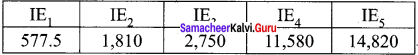(a) phosphorus
(b) sodium
(c) aluminium
(d) silicon table
(c) aluminium

11th Chemistry 3rd Lesson Question 7.
In the third period, the first ionization potential is of the order …………..
(a) Na > Al > Mg > Si > P
(b) Na < Al < Mg < Si < P
(c) Mg > Na > Si > P > Al
(d) Na< Al < Mg < Si < P
(b) Na < Al < Mg < Si < P

11th Chemistry Chapter 3 Question 8.
Identify the wrong statement ……………..
(a) Among st the iso electronic species, smaller the positive charge on cation, smaller is the ionic radius
(b) Among-st iso electric species greater the negative charge on the anion, larger is the ionic radius
(c) Atomic radius of the elements increases as one moves down the first group of the periodic table
(d) Atomic radius of the elements decreases as one moves across from left to right in the 2nd period of the periodic table.
(a) Among-st the iso electronic species, smaller the positive charge on cation, smaller is the ionic radius

Samacheer Kalvi Guru 11th Chemistry Question 9.
Which one of the following arrangements represent the correct order of least negative to most negative electron gain enthalpy?
(a) Al< O<C< Ca< F
(b) Al < Ca<O< C< F
(c) C < F < O < Al < Ca
(d) Ca < Al < C < O < F
(d) Ca < Al < C < O < F

Samacheer Kalvi Class 11 Chemistry Solutions Question 10.
The correct order of electron gain enthalpy with negative sign of F, Cl, Br and I having atomic number 9, 17, 35 and 53, respectively is ………..
(a) J > Br > Cl >F
(b) F > Cl > Br >I
(c) Cl > F > Br >I
(d) Br > I > Cl > F
(c) Cl > F > Br > I

Samacheer Kalvi 11 Chemistry Solutions Question 11.
Which one of the following is the least electro negative element?
(a) Bromine
(b) Chlorine
(c) Iodine
(d) Hydrogen
(d) Hydrogen.
Solution:
Hydrogen is the least electro negative element. Since electro negativity increases across the period from left to right. Hydrogen is the first element and it has less electro negativity and down the group electro negativity decreases.

Samacheer Kalvi 11th Chemistry Chapter 1 Solutions Question 12.
The element with positive electron gain enthalpy is ……….
(a) hydrogen
(b) sodium
(c) argon
(d) fluorine
(c) argon
Solution:
Argon has completely filled configuration. So addition of the electron is not possible and has positive electron gain enthalpy.

11th Chemistry 3rd Chapter Question 13.
The correct order of decreasing electro negativity values among the elements X, Y, Z and A with atomic numbers 4, 8, 7 and 12 respectively –
(a) Y > Z > X > A
(b) Z > A > Y > X
(c) X > Y > Z > A
(d) X > Y >A >Z
(a) Y > Z > X > A

Periodic Classification Of Elements Class 11 Question 14.
Assertion : Helium has the highest value of ionization energy among all the elements known Reason: Helium has the highest value of electron affinity among all the elements known –
(a) Both assertion and reason are true and reason is correct explanation for the assertion
(b) Both assertion and reason are true but the reason is not the correct explanation for the assertion
(c) Assertion is true and the reason is false
(d) Both assertion and the reason are false
(c) Assertion is true and the reason is false

Periodic Classification Of Elements Class 11 Notes Pdf Question 15.
The electronic configuration of the atom having maximum difference in first and second ionization energies is ……….
(a) 1s2, 2s2, 2p6, 3s1
(b) 1s2, 2s2, 2p6, 3s2
(c) 1s2, 2s2, 2p6, 3s2, 3s2, 3p6, 4s1
(d) 1s2, 2s2, 2p6, 3s2, 3p1
(a) 1s2, 2s2, 2p6, 3s1

Chemistry Chapter 3 Answers Question 16.
Which of the following is second most electro negative element?
(a) Chlorine
(b) Fluorine
(c) Oxygen
(d) Sulphur
(a) Chlorine

Periodic Classification Of Elements Class 11 Questions And Answers Question 17.
IE1  and IE2 of Mg are 179 and 348 k cal mol-1 respectively. The energy required for the reaction
Mg → Mg2+ + 2e is ……..
(a) +169 kcal mol-1
(b) -169 kcal mol-1
(c) +527 kcal mol-1
(d) -527 kcal mol-1
(c) +527 kcal mol-1

Class 11 Chemistry Chapter 3 Important Questions With Answers Question 18.
In a given shell the order of screening effect is …………..
(a) s > p > d > f
(b) s > p > f > d
(c) f > d > p > s
(d) f > p > s > d
(a) s > p > d > f

Samacheerkalvi.Guru 11th Chemistry Question 19.
Which of the following orders of ionic radii is correct?
(a) H > H+ > H
(b) Na+ > F“ > O
(c) F > O2- > Na+
(d) None of these
(d) None of these

Samacheer Kalvi.Guru 11th Chemistry Question 20.
The first ionization potential of Na, Mg and Si are 496, 737 and 786 kJ mol-1 respectively. The ionization potential of Al will be closer to
(a) 760 kJ mol-1
(b) 575 kJ mol-1
(c) 801 kJ mol-1
(d) 419 kJ mol-1
(b) 575 kJ mol-1

Class 11 Chemistry Samacheer Solutions Question 21.
Which one of the following is true about metallic character when we move from left to right in a period and top to bottom in a group?
(a) Decreases in a period and increases along the group
(b) Increases in a period and decreases in a group
(c) Increases both in the period and the group
(d) Decreases both in the period and in the group
(a) Decreases in a period and increases along the group

11th Chemistry Solutions Samacheer Kalvi Question 22.
How does electron affinity change when we move from left to right in a period in the periodic table?
(a) Generally increases
(b) Generally decreases
(c) Remains unchanged
(d) First increases and then decreases
(a) Generally increases.

Samacheer Kalvi Guru 11 Chemistry Question 23.
Which of the following pairs of elements exhibit diagonal relationship?
(a) Be and Mg
(b) Li and Mg
(c) Be and B
(d) Be and Al
(d) Be and Al

II. Write brief answer to the following questions

Samacheer Kalvi 11th Chemistry Solution Question 24.
Define modern periodic law.
The modem periodic law states that, “The physical and chemical properties of the elements are periodic function of their atomic numbers.”

Question 25.
What are isoelectronic ions? Give examples.
There are some ions of different elements having the same number of electrons are called isoelectronic ions.
Example:
Na+, Mg2+, Al3+, F , O2- and N3-

Question 26.
What is effective nuclear charge?
The net nuclear charge experienced by valence electrons in the outermost shell is called the effective nuclear charge.
Zeff = Z – S
Where,
Z = Atomic number
S = Screening constant calculated by using Slater’s rules.

Question 27.
Is the definition given below for ionization enthalpy is correct?
“Ionization enthalpy is defined as the energy required to remove the most loosely bound electron from the valence shell of an atom”
No. It is not correct. The accurate and absolute definition is as follows:
Ionization energy is defined as the minimum amount of energy required to remove the most loosely bound electron from the valence shell of the isolated neutral gaseous atom in its ground state.

Question 28.
Magnesium loses electrons successively to form Mg+, Mg2+ and Mg3+ ions. Which step will have the highest ionization energy and why?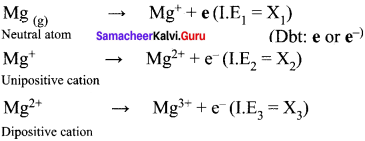• The third step will have the highest ionization energy. I.E3>I.E2>I.E1
• Because from a neutral gaseous atom, the electron removal is easy and less amount of energy is required. But from a di positive cation, there will be more number of protons than the electrons and there is more forces of attraction between the nucleus and electron. So the removal of electron in a di positive cation, becomes highly difficult and more energy is required.

Question 29.
Define electro negativity.
Electro negativity is the relative tendency of an element present in a covalently bonded molecule, to attract the shared pair of electrons towards itself.

Question 30.
How would you explain the fact that the second ionization potential is always higher than first ionization potential?

• Second ionization potential is always higher than first ionization potential.
• Removal of one electron from the valence orbit of a neutral gaseous atom is easy so first ionization energy is less. But from a uni positive ion, removal of one more electron becomes difficult due to the more forces of attraction between the excess of protons and less number of electrons.
• Due to greater nuclear attraction, second ionization energy is higher than first ionization energy.

Question 31.
Energy of an electron in the ground state of the hydrogen atom is -2.18 x 10-18 J. Calculate the ionization enthalpy of atomic hydrogen in terms of kJ mol-1.
Energy of an electron in the ground state of the hydrogen atom = -2.18 x 10-18 J
H → H+ + e
Energy required to ionize 1 mole of hydrogen atoms, we multiply by the Avogadro constant.
E = 2.18 x 10-18 x 6.023 x 1023
= 13.123 x 105 J mol-1
I.E = +1312 K J mol-1

Question 32.
The electronic configuration of an atom is one of the important factor which affects the value of ionization potential and electron gain enthalpy. Explain.

• Electronic configuration of an atom affects the value of ionization potential and electron gain enthalpy.
• Half filled valence shell electronic configuration and completely filled valence shell electronic configuration are more stable than partially filled electronic configuration.
• For e.g. Beryllium (Z = 4) 1s2 2s2 (completely filled electronic configuration)
Nitrogen (Z = 7) 1s2  2s2  2px1  2py2pz1 (half filled electronic configuration) Both beryllium and nitrogen have high ionization energy due to more stable nature.
• In the case of beryllium (1s2 2s2), nitrogen (1s2 2s2 2p3) the addition of extra electron will disturb their stable electronic configuration and they have almost zero electron affinity.
• Noble gases have stable ns2 np6 configuration and the addition of further electron is unfavorable and they have zero electron affinity.

Question 33.
In what period and group will an element with Z = 118 will be present?
The element with atomic number Z = 118 is present in 7th period and 18th group.

Question 34.
Justify that the fifth period of the periodic table should have 18 elements on the basis of quantum numbers.
Fifth period of the periodic table have 18 elements. 5th period starts from Rb to Xe (18 elements). 5th period starts with principal quantum number n = 5 and 1 = 0, 1,2,3 and 4. When n = 5, the number of orbitals = 9.
1 for 5s
5 for 4d
3 for 5p
Total number of orbitals = 9.
Total number of electrons that can be accommodated in 9
orbitals = 9 x 2 = 18. Hence the number of elements in 5th period is 18.

Question 35.
Elements a, b, c and d have the following electronic configurations:
a : 1s2, 2s2, 2p6
b : 1s2, 2s2, 2p6, 3s2, 3p1
c : 1s2, 2s2, 2p3s2,3p6
d : 1s2, 2s2, 2p1
Which elements among these will belong to the same group of periodic table?

1.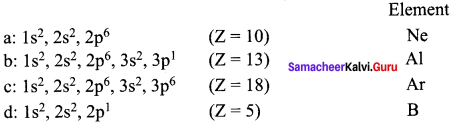2. In the above elements, Ne and Ar belong to same group (Noble gases – 18th group).
3. Al and B belong to the same group (13th group).

Question 36.
Give the general electronic configuration of lanthanides and actinides?

• The electronic configuration of lanthanides is 4f1-14 5d0-16s2.
• The electronic configuration of actinides is 5f1-14 6d0-1 7s2.

Question 37.
Why halogens act as oxidizing agents?
Halogens act as oxidizing agents. Their electronic configuration is ns2 np5. So all the halogens are ready to gain one electron to attain the nearest inert gas configuration. An oxidizing agent is the one which is ready to gain an electron. So all the halogens act as oxidizing agents. Also halogens are highly electro negative with low dissociation energy and high negative electron gain enthalpies. Therefore, the halogens have a high tendency to gain an electron. Hence they act as oxidizing agents.

Question 38.
Mention any two anomalous properties of second period elements.

• In the 1st group, lithium forms compounds with more covalent character while the other elements of this group form only ionic compounds.
• In the 2nd group, beryllium forms compounds with more covalent character while the other elements of this family form only ionic compounds.

Question 39.
Explain the Pauling’s method for the determination of ionic radius.
1. Ionic radius is defined as the distance from the center of the nucleus of the ion up-to which it exerts its influence on the electron cloud of the ion.
2. Ionic radius of uni-univalent crystal can be calculated from the inter-ionic distance between the nuclei of the cation and anion.
3. Pauling assumed that ions present in a crystal lattice are perfect spheres and they are in contact with each other, therefore
d = rC+ + rA ………(1)
Where, d = distance between the center of the nucleus of cation C+ and the anion A-
4. Pauling assumed that the radius of the ion having noble gas configuration (Na+ and F having 1s2, 252, 2p6 configuration) is inversely proportional to the effective nuclear charge felt at the periphery of the ion.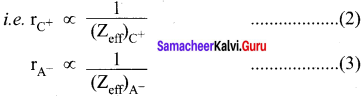Where Zeff is the effective nuclear charge
Zeff = Z – S
5. Dividing the equation (2) by (3)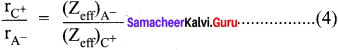On solving equation (1) and (4), the values of rC+ and rA can be obtained.

Question 40.
Explain the periodic trend of ionization potential.
(a) The energy required to remove the most loosely held electron from an isolated gaseous atom is called as ionization energy.
(b) Variation in a period:
Ionization energy is a periodic property. On moving across a period from left to right, the ionization enthalpy value increases. This is due to the following reasons.

• Increase of nuclear charge in a period
• Decrease of atomic size in a period

Because of these reasons, the valence electrons are held more tightly by the nucleus. Therefore, ionization enthalpy increases.

(c) Variation in a group:
As we move from top to bottom along a group, the ionization enthalpy decreases. This is due to the following reasons.

• A gradual increase in atomic size
• Increase of screening effect on the outermost electrons due to the increase of number of inner electrons.

Hence, ionization enthalpy is a periodic property.

Question 41.
Explain the diagonal relationship.

• On moving diagonally across the periodic table, the second and the third period elements show certain similarities.
• Even though the similarity is not same as we see in a group, it is quite pronounced in the following pair of elements.
•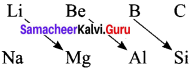• The similarity in properties existing between the diagonally placed elements is called “diagonal relationship”.

Question 42.
Why the first ionization enthalpy of sodium is lower than that of magnesium while its second ionization enthalpy is higher than that of magnesium?
The 1st ionization enthalpy of magnesium is higher than that of Na due to higher nuclear charge and slightly smaller atomic radius of Mg than Na. After the loss of first electron, Na+ formed has the electronic configuration of neon (2,8). The higher stability of the completely filled noble gas configuration leads to very high second ionization enthalpy for sodium. On the other hand, Mg+ formed after losing first electron still has one more electron in its outermost (3 s) orbital. As a result, the second ionization enthalpy of magnesium is much smaller than that of sodium.

Question 43.
By using Pauling’s method calculate the ionic radii of K+ and Cl ions in the potassium chloride crystal. Given that dK+cl = 3.14 Å
Given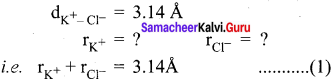We know that,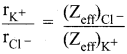(Zeff)Cl = Z – S
= 17 – [(0.35 x 7) + (0.85 x 8) + (1 x 2)]
= 17 – 11.25 = 5.75
(Zeff)K+ = Z – S
= 19 – [(0.35 x 7) + (0.85 x 8) + (1 x 2)]
= 19 – 11.25 = 7.75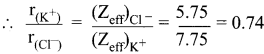r(K+) = 0.74 r(Cl)
Substitute the value of r(K+) in equation (1)
0.74 r(Cl) + r(Cl) = 3.14 Å
1.74 r(Cl) = 3.14Å1.74 = 1.81 Å.

Question 44.
Explain the following, give appropriate reasons.

1. Ionization potential of N is greater than that of O
2. First ionization potential of C-atom is greater than that of B-atom, where as the reverse is true for second ionization potential.
3. The electron affinity values of Be, Mg and noble gases are zero and those of N (0.02 eV) and P (0.80 eV) are very low
4. The formation of F (g) from F(g) is exothermic while that of O2-(g) from O (g) is endothermic.

1. N (Z = 7) 1s2 2s2 2px1 12py1 2pz1. It has exactly half filled electronic configuration and it is more stable. Due to stability, ionization energy of nitrogen is high.
O (Z = 8) 1s2 2s2 2px1 2py1 2pz1. It has incomplete electronic configuration and it requires less ionization energy.
I.E1 N > I.E1O

2. C (Z = 6) 1s2s2 2px1 2py1. The electron removal from p orbital is very difficult. So carbon has highest first ionization potential. B (Z = 5) 1s2 2s2 2p1. In boron nuclear charge is less than that of carbon, so boron has lowest first ionization potential.
I.E1 C > I.EB
But it is reverse in the case of second ionization energy. Because in case of B+ the electronic configuration is 1s2 2s2, which is completely filled and it has high ionization energy. But in C+ the electronic configuration is 1s2 2s2 2p1, one electron removal is easy so it has low ionization energy.
I.EB > I.EC

3. Be (Z = 4) 1s2 2s2
Mg (Z = 12) 1s2 2s2 2p6 3s2
Noble gases has the electronic configuration of ns2 np6. All these are completely filled and are more stable. For all these elements Be, Mg and noble gases, addition of electron is unfavorable and so they have zero electron affinity.

Nitrogen (Z = 7) 1s2s2px1 2py1 2pz1. It has half filled electronic configuration. So addition of electron is unfavorable and it has very low electron affinity value of 0.02 eV. Phosphorus (Z = 15) 1s2 2s2 2p6 3s2 3px1 3py1 3pz1. It also has half filled electronic configuration. Due to the symmetry and more stability, it has very low electron affinity value of 0.80 eV.

4. F(g) + e → F(g) exothermic
F (Z = 9) 1s2 2s2 2p5. It is ready to gain one electron to attain the nearest inert gas configuration. By gaining one electron, energy is released, so it is an exothermic reaction.
O(g) + 2e → O2-(g) endothermic
O (Z = 8) 1s2 2s2 2px1 2py1 2pz1. It is the small atom with high electron density. The first electron affinity is negative because energy is released in the process of adding one electron to the neutral oxygen atom. Second electron affinity is always endothermic (positive) because the electron is added to an ion which is already negative, therefore it must overcome the repulsion.

Question 45.
What is screening effect? Briefly give the basis for Pauling’s scale of electro negativity. Screening effect:
The repulsive force between inner shell electrons and the valence electrons leads to a decrease in the electrostatic attractive forces acting on the valence electrons by the nucleus. Thus the inner shell electrons act as a shield between the nucleus and the valence electrons. This effect . is called shielding effect (or) screening effect.

Pauling’s scale:

• Electro negativity is the relative tendency of an element present in a covalently bonded molecule to attract the shared pair of electrons towards itself.
• Pauling assigned arbitrary value of electronegativities for hydrogen and fluorine as 2.2 and 4, respectively.
• Based on this the electronegativity values for other elements can be calculated using the following expression.
(XA-XB) = 0.182 √EAB – (EAA EBB)
Where EAB , EAA and EBB are the bond dissociation energies of AB, A2 and B2 molecules respectively.
XA and XB are electronegativity values of A and B.

Question 46.
State the trends in the variation of electro negativity in period and group.
Variation of electron negativity in a period:
The electro negativity increases across a period from left to right. Since the atomic radius decreases in a period, the attraction between the valence electron and the nucleus increases. Hence the tendency to attract shared pair of electrons increases. Therefore, electro negativity increases in a period.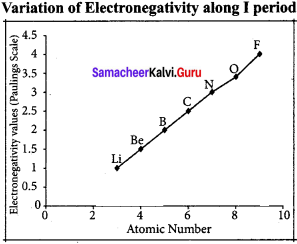Variation of electro negativity in a group:
The electro negativity decreases down a group. As we move down a group, the atomic radius increases and the nuclear attractive force on the valence electron decreases. Hence electro negativity decreases in a group.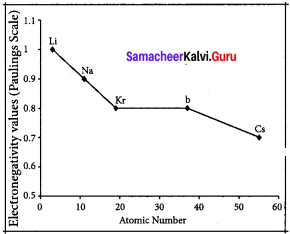Samacheer Kalvi 11th Chemistry Periodic Classification of Elements  In-Text Question – Evaluate your self

Question 1.
What is the basic difference in approach between Mendeleev’s periodic table and modern periodic table?
The main basic difference between Mendeleev’s periodic table and modem periodic table is that first one is constructed on the basis of atomic weight and the later is constructed on the basis of atomic number.

Question 2.
The element with atomic number 120 has not been discovered so far. What would be the IUPAC name and the symbol for this element? Predict the possible electronic configuration of this element.
Atomic number : 120
IUPAC temporary symbol : Unbinilium
IUPAC temporary symbol : Ubn
Possible electronic configuration : [Og] 8s2

Question 3.
Predict the position of the element in periodic table satisfying the electronic configuration (n – 1 )d2 ns2 where n = 5?
Electronic Configuration : (n – 1 )d2 ns2
for n = 5, the electronic configuration is,
1s2 2s2 2p6 3s2 3p6 4s2 3d10 4p6 4d2 5s2
Atomic number : 40
4th group 5th period (d block element) = Zirconium

Question 4.
Using Slater’s rule calculate the effective nuclear charge on a 3p electron in aluminium and chlorine. Explain how these results relate to the atomic radii of the two atoms.
Electronic Configuration of Aluminium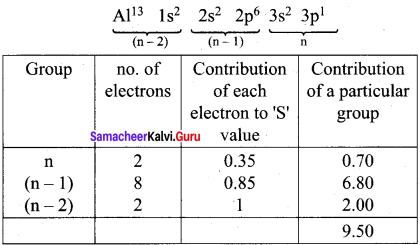Effective nuclear charge = Z – S = 13 – 9.5
(Zeff)Al = 3.5
Electronic Configuration of chlorine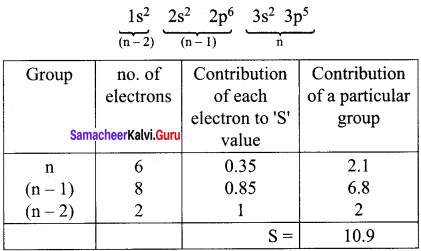Effective nuclear charge = Z- S = 17 – 10.9
(Zeff)Cl = 6.1
(Zeff)Cl > (Zeff)Cl and hence rCl< rAl

Question 5.
A student reported the ionic radii of iso electronic species X3+ , Y2+ and Z as 136 pm, 64 pm and 49 pm respectively. Is that order correct? Comment.
X3+, Y2+, Z are iso electronic.
∴ Effective nuclear charge is in the order
(Zeff)Cl < (Zeff)YY2+ < (Zeff)X3+ and hcnce, ionic radii should be in the order rZ > rY2+ > rX3+
∴ The correct values are: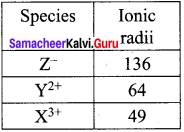Question 6.
The first ionisation energy (IE1) and second ionisation energy (IE2) of elements X, Y and Z are given below.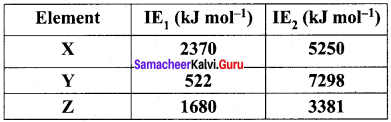Which one of the above elements is the most reactive metal, the least reactive metal and a noble gas?
Noble gases:
Ioniation energy ranging from 2372 KJmol-1 to 1037 kJ mol-1. For element X, the IE1 value is in the range of noble gas, moreover for this element both IE1 and IE2 are higher and hence X is the noble gas. For Y, the first ionisation energy is low and second ionisation energy is very high and hence Y is most reactive metal.
For Z, both IE1 and IE2 are higher and hence it is least reactive.

Question 7.
The electron gain enthalpy of chlorine is 348 kJ mol-1. How much energy in kJ is released when 17.5 g of chlorine is completely converted into Cl ions in the gaseous state?
Cl(g)+ e → Cl(g)
∆H = 348 kJ mol-1
For one mole (35.5g) 348 kJ is released.
∴ For 17.5g chlorine,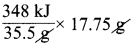energy leased.
∴ The amount of energy released = 3482 = 174 kJ.

### Samacheer Kalvi 11th Chemistry Periodic Classification of Elements  Additional Questions

Question 1.
The chemical symbol of carbon and cobalt are
(a) Ca and CO
(b) Ca and Cl
(c) C and CO
(d) Cr and Cb
(c) C and CO

Question 2.
Consider the following statements.
(i) The chemical symbol of nickel is N.
(ii) An element is a material made up of different kind of atoms.
(iii) The physical state of bromine is liquid.
Which of the above statement is/are not correct?
(a) (i) and (iiii)
(b) (iii) only
(c) (ii) and (iii)
(d) (i) and (ii)
(d) (i) and (ii)

Question 3.
Match the list-I and list-II using the correct code given below the list.
List – I
A. Jewels
B. Bolts and cot
C. Table salt
D. Utensils

List – II
1. Sodium chloride
2. Copper
3. Gold
4. Iron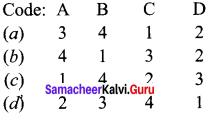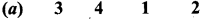Question 4.
The law of triads is not obeyed by
(a) Ca, Sr, Ba
(b) Cl, Br, I
(c) Li, Na, K
(d) Be, B, C
(d) Be, B, C

Question 5.
The law of triads is obeyed by
(a) Fe, CO, Ni
(b) C, N, O
(c) He, Ne, Ar
(d) Al, Si, P
(a) Fe, CO, Ni

Question 6.
Match the list-I and list-II using the code given below the list.
List-I
B. Law of octaves
C. First periodic law
D. Modem periodic law

List-II
1. Chancourtois
2. Henry Moseley
3. Newland
4. Johann Dobereiner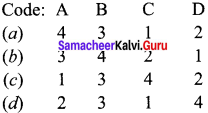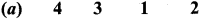Question 7.
Consider the following statements.
(i) In Chancourtois classification, elements differed from each other in atomic weight by 16 or multiples of 16 fell very nearly on the same vertical line.
(ii) Mendeleev’s periodic law is based on atomic weight.
(iii) Mendeleev listed the 117 elements known at that time and are arranged in the order of atomic numbers.
Which of the following statement is/are not correct?
(a) (i) only
(b) (ii) and (iii)
(c) (iii) only
(d) (i),(ii), (iii)
(c) (iii) only

Question 8.
Which of the following elements were unknown at that time of Mendeleev?
(a) Na, Mg
(b) Fe, CO
(c) K, Cu
(d) Ga, Ge
(d) Ga, Ge

Question 9.
Consider the following statements.
(i) Position of hydrogen could not be made clear.
(ii) Isotopes find correct place in Mendeleev’s periodic table.
(iii) Mendeleev’s periodic table could not explain the variable valencies of elements.
Which of the above statement is/are not correct?
(a) (i) only
(b) (i) and (iii)
(c) (ii) only
(d) (i), (ii), (iii)
(c) (ii) only

Question 10.
According to modem periodic law, the physical and chemical properties of the elements are periodic functions of their
(a) atomic volume
(b) atomic numbers
(c) atomic weights
(d) valency
(B) atomic numbers

Question 11.
Which period contain 32 elements?
(a) Period 1
(b) Period 4
(c) Period 5
(d) Period 6
(d) Period 6

Question 12.
There are horizontal rows of the periodic table known as
(a) groups
(b) periods
(c) families
(d) chalcogens
(b) periods

Question 13.
The shortest period contains elements.
(a) H, He
(b) Li, Be
(c) B, C
(a) H, He

Question 14.
The longest form of periodic table was constructed by
(a) Dmitri Mendeleev
(b) Henry Moseley
(c) Lothar Meyer
(d) New lands
(b) Henry Moseley

Question 15.
Match the list-I and list-II using the correct code given below the list.
List – I
Z = 100
Z = 101
Z = 102
Z = 103

List – II
1. Mendelevium
2. Lawrencium
3. Fermium
4. Nobelium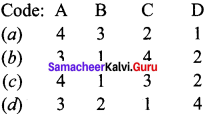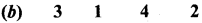Question 16.
Which one of the following is the first transition series?
(a) Sc
(b) Zn
(c) Ti
(d) Cu
(a) Sc

Question 17.
(a) 4th period
(b) 7th period
(c) 6th period
(d) 31 period
(b) 7th period

Question 18.
Which one of the following is called halogen family?
(a) Group 17
(b) Group 16
(c) Group 1
(d) Group 2
(a) Group 17

Question 19.
Group 16 constitutes family.
(a) halogen
(b) nobel gas
(c) chalcogen
(d) alkali metals
(c) chalcogen

Question 20.
Consider the following statements.
(i) The valency of the elements increases from left to right in a period.
(ii) Valency decreases from 7 to I with respect to oxygen.
(iii) The metallic character of the elements decreases across a period.
Which of the above statement is/are not correct?
(a) (i) only
(b) (ii) only
(c) (i) and (iii)
(d) (i) (ii) and (iii)
(b) (ii) only

Question 21
Match the list – I and list -II using the correct code given below the list.
List – I
A. Li
B. Na
C. K
D. Cs

List – II
1. 2,8,8,1
2. 2,1
3. 2,8, 18, 18, 8, 1
4. 2,8, 1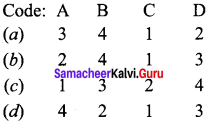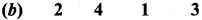Question 22.
What will be the change in valency down the group in the periodic table?
(a) increases
(b) decreases
(c) remains same
(d) zero
(c) remains same

Question 23.
Which one of the following is a metalloid?
(a) N
(b) P
(c) Bi
(d) Sb
(d) Sb

Question 24.
Which one of the following is a metal?
(a) N
(b) Br
(c) Bi
(d) As
(c) Bi

Question 25.
Match the list – I and list – II using the correct code given below the list.
List – I
A. Alkali metal
B. Alkaline earth metals
C. d-block elements
D. p-block elements

List – II
1. ns2 np1-6
2. ns1
3. ns2
4. (n – 1)d1-10 ns0-2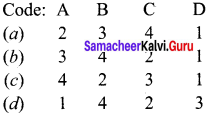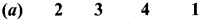Question 26.
Consider the following statements.
(i) Oxidation character increases from left to right in a period.
(ii) Reducing character increases from left to right in a period.
(iii) Metallic character increases from left to right in a period.
Which of the above statement is/are not correct?
(a) (i) only
(b) (i) and (iii)
(c) (ii) and (iii)
(d) (i), (ii), (iii)
(c) (ii) and (iii)

Question 27.
The general electronic configuration of d-block elements is
(a) ns2 nd1-10 10
(b) (n-1)d1-10 ns0-2
(c) (n-2)d1-10 (n – 1)0-2
(d) ns2nd5
(b) (n-1)d1-10 ns0-2

Question 28.
Consider the flowing statements.
(i) d-block elements show variable oxidate states.
(ii) Mostly d-block elements form colourless compounds.
(iii) Mostly d-block elements are diamagnetic due to paired electrons.
Which of the above statement is/are not correct?
(a) (i) only
(b) (ii) only
(c) (i) and (ii)
(d) (ii) and (iii)
(d) (ii) and (iii)

Question 29.
All the s – block and p-block elements excluding l8 group are called elements.
(a) representative
(b) transition
(c) inner – transition
(d) trans uranium
(a) representative

Question 30.
Which of the following is the correct electronic configuration of noble gases?
(a) ns2 npnd10
(b) ns2 np5
(c) ns2 np6
(d) ns2 np3
(c) ns2 np6

Question 31.
Group numbers 13 to 12 in the periodic table are called …………..
(a) inner transition elements
(b) Representative elements
(c) synthetic elements
(d) transition elements
(d) transition elements

Question 32.
Which one of the following is in solid state at room temperature?
(a) Bromine
(b) Mercury
(c) Bismuth
(d) Gallium
(c) Bismuth

Question 33.
Which of the following is not a metalloid (or) semi-metal?
(a) Silicon
(b) Arsenic
(c) Germanium
(d) Sodium
(d) Sodium

Question 34.
Which of the following metal is not in liquid state?
(a) Gallium
(b) Aluminium
(c) Mercury
(d) Calsium
(b) Aluminium

Question 35.
Which of the following is not a periodic property?
(b) Ionization enthaphy
(c) Electron affinity
(d) Oxidation number
(d)Oxidation number

Question 36.
Which of the following property increases as we go down the group in the periodic property?
(a) ionization energy
(b) Electro negativity
(d) Electron affinity

Question 37.
The metallic radius of copper is ………………
(a) 0.99 Å
(b) 1.28 Å
(c) 1.98 Å
(d) 2.56 Å
(5) 1.28 Å

Question 38.
Consider the following statements………………
(i) Atomic radius of elements increases with increase in atomic number as we go down the group.
(ii) Atomic radius of elements increases with increase in atomic number as we go across the period.
(iii) Atomic radius of elements decreases as we go from left to right in a period.
Which of the above statement is/are not correct?
(a) (i) only
(b) (i) and (ii)
(c) (ii) only
(d) (i) and (iii)
(c) (ii) only

Question 39.
Which one of the following is not an iso electronic ion?
(a) Na+
(b) Mg2+
(c) Cl
(d) O2-
(c) Cl

Question 40.
Which one of the following is not an isoelectronic ion?
(a) Al3+
(b) N3-
(c) Mg2+
(d) K+
(d) K+

Question 41.
Which of the following possess almost same properties due to lanthanide contraction?
(a) Zr, HF
(b) Na, K
(c) Zn, Cd
(d) Ag. Au
(a) Zr, HF

Question 42.
Consider the following statements.
(i) Ionization is always an exothermic process.
(ii) Ionization energies always increase in the order I.E1> IE2>I.E3.
(iii) Ionization energy measurements are carried out with atoms in the solid state.
Which of the above statement is/are not correct?
(a) (i) only
(b) (i) and (ii)
(c) (iii) only
(d) (i), (ii) and (iii)
(d) (i), (ii) and (iii)

Question 43.
Statement-I : Ionization enthalpy of Be is greater than that of 13.
Statement-II : The nuclear charge of B is greater than that of Be.
(a) Statement-I and II are correct and statement-II is the correct explanation of statement-I.
(b) Statement-I and II are correct but statement-II is not the correct explanation of statement-I.
(c) Statement-I is correct but statement-II is wrong.
(d) Statement-I is wrong but statement-II is correct.
(b) Statement-I and II are correct but statement-II is not the correct explanation of statement-I.

Question 44.
Statement-I: Ionization enthalpy of nitrogen is greater than that of oxygen.
Statement-lI: Nitrogen has exactly half filled electronic configuration which is more stable than electronic configuration of oxygen.
(a) Statement-I is wrong but statement-II is correct.
(b) Statement-I is correct but statement-II is wrong.
(c) Statement-I and II are correct and statement-TI is the correct explanation of statement-I.
(d) Statement-I and II are correct but statement-II is not the correct explanation of statement-I.
(c) Statement-I and II are correct and statement-II is the correct explanation of statement-I.

Question 45.
Which of the following does not have zero electron gain enthalpy?
(a) Be
(b) Cl
(c) Mg
(d) N
(b) Cl

Question 46.
Which of the following have zero electron gain enthalpy?
(a) Halogens
(b) Noble gases
(c) Chalcogens
(d) Gold
(b) Noble gases

Question 47.
Which of the following have the highest value of electronegativity?
(a) Halogens
(b) Alkali metals
(c) Alkaline earth metals
(d) Transition metals
(a) Halogens

Question 48.
Among all the elements which one has the highest value of electronegativity?
(a) Chlorine
(b) Bromine
(c) Fluorine
(d) Iodine
(c) Fluorine

Question 49.
Among the alkali metals which one form compounds with more covalent character?
(a) Sodium
(b) Potassium
(c) Rubidium
(d) Lithium
(d) Lithium

Question 50.
Which of the following pair is not diagonally related?
(a) Li, Mg
(b) Li, Na
(c) Be, Al
(d) B, Si
(b) Li, Na

Question 51.
In the modern periodic table, the period indicates the value of
(a) atomic number
(b) mass number
(c) principal quantum number
(d) azimuthal quantum number
(c) principal quantum number
Hint:
In the modern periodic table, each period begins with the filling of a new shell. Therefore, the period indicates the value of principal quantum number. Thus, option (c) is correct.

Question 52.
Which of the following statements related to the modern periodic table is incorrect?
(a) The p-block has 6 columns, because a maximum of 6 electrons can occupy all the orbitais in a p-subshell.
(b) The d-block has 8 columns, because a maximum of 8 electrons can occupy all the orbitais in a d-subsheli.
(c) Each block contains a number of columns equal to the number of electrons that can occupy that subshell.
(d) The block indicates value of azimuthal quantum number (1) for the last subshell that received electrons in building up the electronic configuration.
(b)The d-block has 8 columns, because a maximum of 8 electrons can occupy all the orbitais in a d-subshell.

Question 53.
The size of isoelectronic species- F, Ne and Na+ is affected by
(a) nuclear charge (Z)
(b) valence principal quantum number (n)
(c) electron-electron interaction in the outer orbitais
(d) none of the factors because their size is the same
(a) nuclear charge (Z).

Question 54.
Which of the following statements is incorrect in relation to ionization enthalpy?
(a) Ionization enthalpy increases for each successive electron.
(b) The greatest increase in ionization enthalpy is experienced on removal of electrons from core noble gas configuration.
(c) End of valence electrons is marked by a big jump in ionization enthalpy.
(d) Removal of electron from orbitais bearing lower n value is easier than from orbital having high n value.
(d)Removal of electron from orbitais bearing lower n value Is easier than from orbital having high n value.

Question 55.
Considering the elements B, Al, Mg and K, the correct order of their metallic character is:
(a) B > Al >Mg > K
(b) Al > Mg > B > K
(c) Mg > Al > K > B
(d) K > Mg > Al > B
(d) K > Mg > Al> B
Hint:
In a period, metallic character decreases as we move from left to right. Therefore, metallic character of I< Mg and Al decreases in the order: K> Mg > Al. However, within a group, the metallic character, increases from top to bottom. Thus, Al is more metallic than B.Therefore, the correct sequence of decreasing metallic character is K> Mg >Al > B, i.e,
option (d) is correct.

Question 56.
Considering the elements B, C, N, F and Si, the correct order of their non-metallic character is
(a) B>C>Si>N>F
(b) Si>C>B>N>F
(c) F>N>C>B>Si
(d) F>N>C>Si>B
(c) F>N>C>B> Si
Hint:
In a period, the non-metallic character increases from left to right. Thus, among B, C, N and F, non-metallic character decreases in the order: F > N > C> B. However, within a group, non-metallic character decreases from top to bottom. Thus, C is more non-metallic than Si. Therefore, the correct sequence of decreasing non-metallic character is: F> N > C > B > Si, i.e., option (c) is correct.

Question 57.
Considering the elements F, Cl, O and N, the correct order of their chemical reactivity in terms of oxidizing property is
(a) F>Cl>O>N
(b) F>O>Cl>N
(c) Cl>F>O>N
(d) O>F>N>Cl
(b) F>O>Cl>N.
Hint:
Within a period, the oxidizing character increases from left to right. Therefore, among F, O and N,oxidizing power decreases in the order: F> O> N. However, within a group, oxidizing power decreases from top to bottom. Thus, F is a stronger oxidizing agent than Cl. Further because O is more electronegative than Cl, therefore, O is a stronger oxidizing agent than Cl. Thus, overall decreasing order of oxidizing power is: F > O > Cl > N, i.e.,
option (b) is correct.

Question 58.
The highest ionization energy is exhibited by ………………
(a) halogens
(b) alkaline earth metals
(c) transition metals
(d) noble gases
(b) alkaline earth metals

Question 59.
Which of the following is arranged in order of increasing radius?
(a) K+(aq) < Na+(aq) <Li+(aq)
(b) K+(aq)> Na+(aq)> Zn2+(aq)
(c) K+(aq)> Li+(aq) > Na+(aq)
(d) Li+(aq)< Na+(aq) < K+(aq)
(d) Li+(aq)< Na+(aq) < K+(aq)

Question 60.
Among the following elements, which has the least electron affinity?
(a) Phosphorous
(b) Oxygen
(c) Sulphur
(d) Nitrogen
(d) Nitrogen

Question 61.
Which one of the following is isoelectronic with Ne?
(a) N3-
(b) Mg2+
(c) Al3+
(d) All the above
(d) All the above

Question 62.
Which clement has smallest size?
(a) B
(b) N
(c) Al
(d) P
(b) N

Question 63.
In halogens, which of the following decreases from iodine to fluorine?
(a) Bond length
(b) Electronegativity
(c) ionization energy
(d) Oxidizing power
(a) Bond length

Question 64.
What is the electronic configuration of the elements of group 14?
(a) ns2 np4
(b) ns2 np6
(c) ns2 np2
(d) ns2
(c) ns2 np2

Samacheer Kalvi 11th Chemistry Periodic Classification of Elements  2-Mark Questions

Write brief answer to the following questions:

Question 1.
State Johann Dobereiner’s law of triads.
Johann Dobereiner noted that elements with similar properties occur in groups of three which he called triads. It was seen that invariably, the atomic weight of the middle number of the triad was nearly equal to the arithmetic mean of the weights of the other two numbers of the triad.
For e.g.,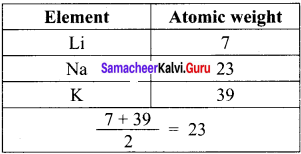Question 2.
Write a note about Chancourtois classification.
in this system, elements that differed from each other in atomic weight by 16 or multiples of 16 fell very nearly on the same vertical line. Elements lying directly under each other showed a definite similarity. This was the first periodic law.

Question 3.
State the New land’s law of octaves.
The Law of octaves states that, “when elements are arranged in the order of increasing atomic weights, the properties of the eighth element are a repetition of the properties of the first element.

Question 4.
State Mendeleev’s periodic law.
This law states that “The physical and chemical properties of elements are a periodic function of their atomic weights.”

Question 5.
Explain about the relationship between the atomic number of an element and frequency of the X-ray emitted from the elements.
Henry Moseley studied the X-ray spectra of several elements and determined their atomic numbers (Z). He noticed that the frequencies of X-ray emitted from the elements concerned could be correlated by the equation
υ−−√ = a(Z – b)
Where, υ Frequency of the X-ray emitted by the element.
a and b = Constants and have same values for all the elements.
Z = Atomic number of the element.

Question 6.
State modern periodic law.
The modem periodic law states that, “The physical and chemical properties of the elements are periodic function of their atomic numbers.”

Question 7.
What are the anomalies of the long form of periodic table?
The long form of periodic table need clarification about the following:

• Position of hydrogen is not defined till now.
• Lanthanides and actinides still find place in the bottom of the table.

Question 8.
Mention the names of the elements with atomic number 101, 102, 109 and 110.
Z = 101  IUPAC  name : Mendelevium
Z = 102  IUPAC  name : Nobelium
Z = 109  IUPAC  name : Meitnerium
Z = 110  IUPAC  name : Darmstadtium

Question 9.
Write a note about the electronic configuration of elements in groups.
A vertical column of the periodic table is called a group. A group consists of a series of elements having similar configuration of the outermost shell. There are 18 groups in the periodic table. it may be noted that the elements belonging to the same group are said to constitute a family. For example, elements of group 17 are called halogen family.

Question 10.
Give the name and electronic configuration of elements of group and 2 group.

• Elements of 1st group are called alkali metals. Their electronic configuration is ns1.
• Elements of 2nd group are called alkaline earth metals. Their electronic configuration is ns2.

Question 11.
Write any two characteristic properties of alkali metals.

• Alkali metals readily lose their outermost electron to form +1 ion.
• Alkali metals are soft metals with low melting and boiling points.

Question 12.
Write any two characteristic properties of alkaline earth metals.

• Alkaline earth metals readily lose their outermost electrons to form +2 ion.
• As we go down the group. their metallic character and reactivity are increased.

Question 13.
Groups from 13 to 1 in the periodic table are called p-block elements. Give reason.

• The elements whose last electron enters into the p-orbital of the outermost shell are having similar properties and thus form a group. The ‘np’ orbital of these elements is being progressively tilled. Hence, these elements are named as p-block elements.
• The groups of 3th to 18th in the periodic table belongs to p-block.

Question 14.
Why noble gases do not show much of chemical reactivity?
Noble gases having closed valence shell configuration as ns2 np6. The valence shell orbitais of noble gases are completely filled by electrons and it is very difficult to alter this stable arrangement by the addition or removal of electrons. Because of these reasons noble gases do not show much of chemical reactivity.

Question 15.
Halogens and chalcogens have highly negative electron gain enthalpies. Why?

• Group 16 (chaicogens) and Group 17 (halogens) are interested to add two or one electrons respectively to attain stable noble gas configuration.
• Because of this interest these elements have highly negative electron gain enthalpies.

Question 16.
What are d-biock elements? Why are they called so?

• The elements whose last electron enters into the d-orbitalof the penultimate shell (n-1) are having similar properties and called as d-block elements.
• The groups of 3 to 12 in the center of the periodic table belongs to d-block.

Question 17.
Elements Zn, Cd and Hg with electronic configuration (n-1)d10 ns2 do not show most of transition elements properties. Give reason.

• Zn, Cd and Hg are having completely filled d-orbitais (d10 electronic configuration).
• They do not have partially filled d-orbitais Like other transition elements. So they do not show much of the transition elements properties.

Question 18.
Why Zn, Cd and Hg are considered as soft metals?

• Zinc, cadmium and mercury are metals with low melting points. This is because they have an especially stable electronic configuration.
• Mercury is so poor at forming metallic bonds that it is liquid at room temperature.
• Zinc and cadmium arc soft metals that oxidize to the +2 oxidation states.

Question 19.
Why d-block elements are called as transition elements?
d-block elements form a bridge between the chemically active metals of s-block elements and the less active elements of groups of 13th and 14th and thus take their familiar name transition elements.

Question 20.
What are f-block elements? how many series are there? Why they are called f-block elements?

• The elements, whose last electron enters into the f-orbital of the ante-penultimate shell (n-2) are having similar properties are called f-block elements. In these elernents (n-2)f orbitais are being filled progressively.
• The two rows of elements placed at the bottom of the periodic table. They are lanthanides and actinides.

Question 21.
Write the electronic configuration of lanthanides and actinides?

• The electronic configuration of lanthanides is 4f1-144 5d0-11 6s2.
• The electronic configuration of actinides is 5f1-14 6d0-17s2.

Question 22.
What are lanthanides and actinides? ,

• In 4f senes, 4f’orbitals arc being progressively filled with electrons, 4f1-14 5d0-1 6s2. These elements lie in 6th period and are called rare earths or lanthanides or lanthanones.
• In 5f series, 5f orbitais are being progressively filled with electrons, 5f16d0-1 7s2. These elements lie in 7th period and are called actinides or actonones.

Question 23.
What are semi-metals? Give example.

• Some elements in the periodic table show properties that are characteristic of both metals and non-metals. These elements are called semi-metals or metalloids.
• Example: Silicon. germanium, arsenic, antimony and tellurium.

Question 24.
What are periodic properties? Give example.
The term periodicity of properties indicates that the elements with similar properties reappear at certain regular intervals of atomic number in the periodic table.
Example:

• Ionisation energy
• Electron affinity
• Electronegativity.

Question 25.
The ionic radius of an ion is the distance between the center of the ion and the outermost point of its electron cloud.

Question 26.
Cationic radius is smaller than its corresponding neutral atom. Justify this statement.

• When an neutral atom lose one or more electrons it forms cation.
Na → Na+ + e
• The radius of this cation (rNa+)is decreased than its parent atom (rNa).
• When an atom is charged to cation, the number of nuclear charge becomes greater than the number of orbital electrons. Hence the remaining electrons are more strongly attracted by the nucleus. Hence the cationic radius is smaller than its corresponding neutral atom.

Question 27.
Anionic radius is higher than the corresponding neutral atom. Give reason.
When an atom gain one or more electrons it forms anion. During the formation of anion, the number of orbital electrons become greater than the nuclear charge. Hence, the electrons are not strongly attracted by the lesser number of nuclear charges. Hence anionic radius is higher than the corresponding neutral atom.

Question 28.
What are iso electronic ions? Give example.
There are some ions of different elements having the same number of electrons are called isoelectronic ions.
Example: Na+ Mg2+, Al3+, F, O2-, N3-

Question 29.
Define ionization energy. Give its unit.
The energy required to remove the most loosely held electron from an isolated gaseous atom is called ioniiation energy.
M(g) + energy M+(g) + electron
The unit of ionization energy is KJ mole-1.

Question 30.
Ionization energy of beryllium is greater than the ionization energy of boron. Why?
Be (Z= 4) 1s2 2s2. it has completely filled valence electrons, which requires high IE1.
B (Z =5) 1s2 2s2 2p1. It has incompletely filled valence electrons, which requires comparatively
less IE1 Hence I.E1 Be > I.E1 B.

Question 31.
Ionization energy of nitrogen is greater than the ionization energy of oxygen. Give reason.
7N 1s2 2s1 2p3 (or)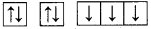. Nitrogen has exactly half filled valence electrons, which requires high I.E1.
8O 1s2 2s2 2p4 (or)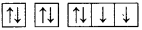. Oxygen has incomplete valence shell electrons, which requires comparatively less I.E1.
I.E1N>I.E1O

Question 32.
Define electron gain enthalpy or electron affinity. Give its unit.
The electron gain enthalpy of an element is the amount of energy released when an electron is added to the neutral gaseous atom.
A + electron → A + energy (E.A)
Unit of electron affinity is KJ mole.

Question 33.
Electron gain enthalpy of F ¡s less negative than Cl. Why?
When an electron is added to F, the added electron goes to the L shell (n = 2). As the ‘L’ shell possess smaller region of space, the added electron feels significant repulsion from the other electrons present in this level.
E.A of F = -328 KJ mole-1
E.A of Cl = -349 KJ mole-1

Question 34.
Electron affinity of oxygen is less negative than sulphur. Justify this statement.
When an electron is added to oxygen, the added electron goes to the ‘L’ shell (n 2). As the ‘L’ shell possess smaller region of space, the added electron feels significant repulsion from the other electrons present in this level.
E.A of O = – 141 KJ mole.
E.A of S = – 200 KJ mole.

Question 35.
Explain about the factors that affect electro negativity.

• Effective nuclear charge:
As the nuclear charge increases, electro negativity also increases along the periods.
The atoms in smaller size will have larger electronegativity.

Question 36.
Explain about periodic variation of electro negativity across a period.
As we move from left to right in a period, electro negativity increases. This is due to the following reasons:

• Nuclear charge increases in a period
• Atomic size decrease in a period

Halogens have the highest value of electro negativity in their respective periods.

Question 37.
Explain about the period variation of electro negativity along a group.
As we move down from top to bottom in a group, electro negativity decreases due to increased atomic radius. Fluorine has the highest value of clectro negativity among all the elements.

Question 38.
Define valency. How is it determined?
The valency of an element may be defined as the combining capacities of elements. The electrons present in the outermost shell are called valence electrons and these electrons determine the valency of the atom.

Question 39.
What is the basic difference in approach between Mendeleev’s periodic law and the modern periodic law?
The basic difference in approach between Mendeleev’s periodic law and modern periodic law is the change in basis of classification of elements from atomic weight to atomic number.

Question 40.
On the basis of quantum numbers, justify that the sixth period of the periodic table should have 32 elements.
The sixth period corresponds to sixth shell. The orbitais present in this shell are 6s, 4f. 5p and 6d. The maximum number of electrons which can be present in these sub-shell is 2 + 14 + 6 + 10 = 32. Since the number of elements in a period corresponds to the number of electrons in the shells, the sixth period should have a maximum of 32 elements.

Question 41.
Why do elements in the same group have similar physical and chemical properties?
The elements in a group have same valence shell electronic configuration and hence have similar physical and chemical properties.

Question 42.
How do atomic radius vary in a period and in a group? How do you explain the variation.
Within a group atomic radius increases down the group Reason :
This is due to continuous increases in the number of electronic shells or orbit numbers in the structure of atoms of the elements down a group.

Variation across period:
From left to right across a period atomic radii generally decreases due to increase in effective nuclear charge from left to right across a period.

Question 43.
Explain why cation are smaller and anions are larger ¡n radii than their parent atoms?
A cation is smaller than the parent atom because it has fewer electrons while its nuclear charge remains the same. The size of anion will be larger than that of parent atom because the addition of one or more electrons would result in increased repulsion among the electrons and a decrease in effective nuclear charge.

Question 44.
What is basic difference between the terms electron gain enthalpy and electronegativity?
Electron gain enthalpy refers to tendency of an isolated gaseous atom to accept an additional electron to form a negative ion. Whereas electronegativity refers to tendency of the atom of an clement to attract shared pair of electrons towards it in a covalent bond.

Question 45.
Would you expect the first ionization enthalpies of two isotopes of the same element to be the same or different? Justify your answer.
Ionization enthalpy, among other things, depends upon the electronic configuration (number of electrons) and nuclear charge (number of protons). Since isotopes of an element have the same electronic configuration and same nuclear charge, they have same ionization enthalpy.

Question 46.
Write the general electronic configuration of s-, p-, d-, and f-block elements?

• s-block elements : ns1-2 where n 2 – 7.
• p-block elements : ns2 np1-6 where n = 2 – 6.
• d-block elements : (n – 1) d1-0 ns0-2 where n = 4 – 7.
• f-block elements : (n – 2) f0-14 (n – 1) d0-1 ns2 where n = 6 – 7.

Samacheer Kalvi 11th Chemistry Periodic Classification of Elements 3-Mark Questions

Question 1.
Why there is a need for classification of elements?

• Classification is a fundamental and essential process in our day-to-day life for the effective utilization of resources, daily events and materials.
• In such a way, for the effective utilization of discovered elements becomes fundamentally essential process.
• The periodic classification of the elements is one of the outstanding contributions to the progress of chemistry.

Question 2.
Prove that the halogens, chlorine, bromine and iodine follow the law of triads.
When the halogens, chlorine, bromine and iodine are placed on below the others, they had similar properties. The atomic weight of bromine was close to the average of the atomic weights of chlorine and iodine.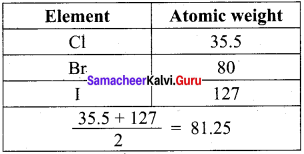Question 3.
What are the salient features of New land’s law of octaves?

1. This law is quite well for lighter elements but not supported to heavier elements.
2. Elements were arranged in increasing atomic masses without taking an account on the properties of elements.
3. This law was seemed to be applicable only for elements upto calcium.

Question 4.
How the properties of Eka – silicon was related to germanium?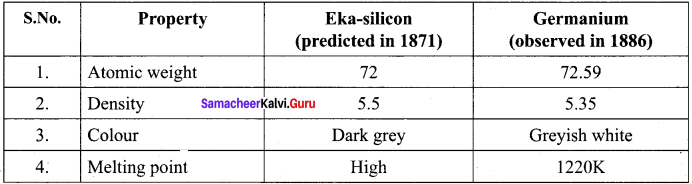Question 5.
Compare the properties of Eka – aluminium and gallium.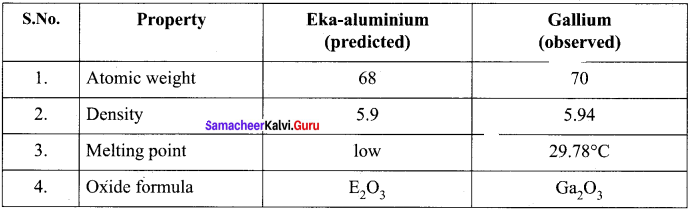Question 6.
How Moseley determined the atomic number of an element using X-rays?

• Henry Moseley studied the X-ray spectra of several elements and determined their atomic numbers (Z).
• He discovered a correlation between atomic number and the frequency of X-rays generated by bombarding an clement with high energy of electrons.
• Moseley correlated the frequency of the X-ray emitted by an equation as,
v = a (Z – b)
Where υ = Frequency of the X-rays emitted by the elements.
a and b = Constants.
• From the square root of the measured frequency of the X-rays emitted, he determined the atomic number of the element.

Question 7.
What are the reasons behind the Moseley’s attempt in finding atomic number?

• The number of electrons increases by the same number as the increase in the atomic number.
• As the number of electrons increases, the electronic structure of the atom changes.
• Electrons in the out cannost shell of an atom (valence shell electrons) determine the chemical properties of the elements.

Question 8.
Draw a simplified form of periods and elements present in modern period table.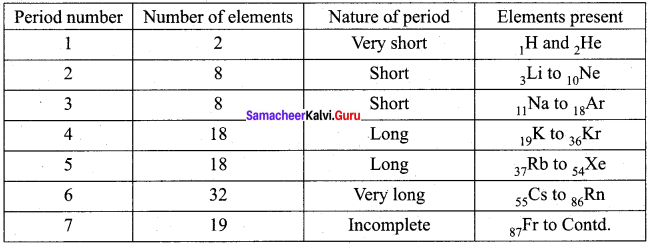Question 9.
Write the electronic configuration of alkali metals 2Li,11Na, 19K, 37Rb, 55Cs and 87Fr.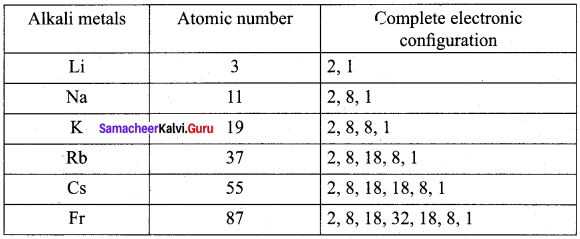Question 10.
Explain about the classification of elements based on electronic configuration.

• The distribution of electrons into orbitais, s, p, d and f of an atom is called its electronic configuration. The electronic configuration of an atom is characterized by a set of four quantum numbers, n, l, m and s. of these the principal quantum number (n) defines the main energy level known as shells.
• The position of an element in the periodic table is related to the configuration of that element and thus reflects the quantum numbers of the last orbital filled.
• The electronic configuration of elements in the periodic table can be studied along the periods and groups separately for the best classification of elements.
• Elements placed in a horizontal row of a periodic table is called a period. There are seven periods.
• A vertical column of the periodic table is called a group. A group consists of a series of elements having similar configuration of the outermost shell. There are 18 groups in periodic table.

Question 11.
Write about the electronic configuration of 1st and 2nd period.
Electronic configuration of t period:
In 1 period only two elements are present. This period starts with the filling of electrons in first energy level, n1. This level has only one orbital as is. Therefore it can accommodate two electrons maximum.

Electronic configuration of 2nd period:
In the 2’ period 8 elements are present. This period starts with filling of electrons in the second energy level, n = 2. In this level four orbitais (one 2s and three 2p) are present. Hence the second energy level can accommodate 8 electrons. Thus, second period has eight elements.

Question 12.
How many elements are there in 4th period? Prove it.
In fourth period, 18 elements are present. In this period electrons are entering into fourth energy level, i.e., n = 4. it starts with the filling of 4s-orbitals. However, after the 4s, but before the 4p orbitais, there are five 3d-orbitais also to be filled. Thus, nine orbitais (one 4s, five 3d and three 4p) have to be filled. These nine orbitais can accommodate (9 x 2 = 18)18 electrons. Hence, period contain 18 elements in it.

Question 13.
How many elements are there in 6th period? Prove it.
In sixth period, 32 elements are present. This period starts with the filling of 6th energy shell, n = 6. There are sixteen orbitais (one 6s, seven 4f, five 4d and three 6p) to be filled. These sixteen orbitais can accommodate 32 (16 × 2 = 32) electrons. Hence, 32 elements are present in sixth period.

Question 14.
What are the two exceptions of block division in the periodic table?
1. Helium has two electrons. Its electronic configuration is 1s2. As per the configuration, it is supposed to be placed in ‘s’ block, but actually placed in s group which belongs to ‘p’ block. Because it has a completely filled valence shell as the other elements present in 18th group. It also resembles with 18th group elements in other properties. Hence helium is placed with other noble gases.

2. The other exception is hydrogen. it has only one s-electron and hence can be placed in group 1. It can also gain an electron to achieve a noble gas arrangement and hence it can behave as halogens (17th group elements). Because of these assumptions, position of hydrogen becomes a special case. Finally, it is placed separately at the top of the periodic table.

Question 15.
Explain about the salient features of metals.

• Metals comprise more than 78% of all known elements. They are present on the left side of the periodic table.
• They are usually solids at room temperature. [Mercury is an exception (Hg-liquid), gallium (303 K) and cesium (302 K) also have very low melting points].
• Metals usually have high melting and boiling points.
• They are good conductors of heat and electricity.
• They are malleable and ductile, and also can be flattened into thin sheets by hammering and drawn into thin wires.

Question 16.
Explain about the characteristics of non-metals.

• Non-metals are located at the top right hand side of the periodic table.
• In a period, as we move from left to right the non-metallic character increases while the metallic character increases as we go down a group.
• Non-metals are usually solids or liquids or gases at room temperature with low melting and low boiling points (Exceptions : boron and carbon).
• They are poor conductors of heat and electricity.
• Most of the non-metallic solids are brittle and are neither malleable nor ductile.

Question 17.
Periodic change in electronic configuration is responsible for the physical and chemical properties of element. Justify this statement.

• The electronic configuration of the elements changes periodically in a period and group as well.
• We could find a pattern in the physical and chemical properties as we go down in a group or move across a period.
• For example : The chemical reactivity is high at the beginning, lower at the middle and increases to a maximum at group 17 in a period.
• The reactivity increases on moving down the group of alkali metals. But the reactivity decreases on moving down the group of halogens.
• Atomic radii increases down the group and decreases across the period.

Question 18.
What is covalent radius? How would you determine the covalent radius of chlorine atom?
The distance between the nuclei of two covalent bonded atoms is known as covaLent distance or inter-nuclear distance. The one – half of this inter-nuclear distance is called covalent radius. The covalent distance (Cl – Cl) of Cl2 molecule is experimentally found as 198 pm (1.98 A). Its covalent radius is 99 pm (0.99 Å).
Cl – Cl Inter nuclear distance = 1.98 Å
∴ rCl = 1.98 / 2=0.99 Å.

Question 19.

• It is defined as one half of the distance between the centers of nuclei of the two adjacent atoms in the metallic crystal.
• The distance between two adjacent copper atoms in solid copper is 2.56 A. Hence, the metallic radius of copper is 1.28 A.

Question 20.
Arrange Na+, Mg2+ and Al3+ in the increasing order of ionic radii. Give reason.
Na+, Mg2+ and Al3+ are iso electronic cations.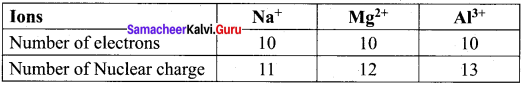The cation with the greater positive charge will have a smaller radius because of the greater attraction of the electrons to the nucleus. Hence the increasing order of ionic radii is,
rNa+ > rMg2+ > rAl3+

Question 21.
Arrange the ions F, O2- and N3- ¡n the increasing order of their ionic radii. Give reason.
F, O2- and N3- are isoelectronic species.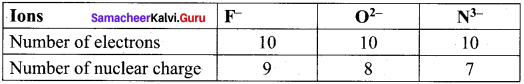The anion with the greater negative charge will have a larger radius because of the lesser attraction of the electrons to the nucleus. Hence the increasing order of ionic radii is,
rNa3 > rO2 > rF

Question 22.
Mention some characteristics of ionization energy.

• Ionization is always an endothermic process. It absorbs energy.
• Ionization energies always increase in the order, I.E1< I.E2<IE3.
• Ionization energy measurements are carried out with atoms in the gaseous state.

Question 23.
Why ionization energy and electron affinity are calculated in gaseous state?

• Inter molecular force can affect the value of ionization energy and electron affinity.
• In gaseous state, there is little inter molecular force in a substance and it can be considered negligible In some cases. So the value of I.E and E.A are almost unaffected if they are calculated for gaseous atoms.
• When we arc talking about ionization energy and electron affinity, we need to consider
atoms and we can find free atoms only when the substance is in gaseous state.

Question 24.
How is screening effect of inner electrons affect ionization energy?

• The electrons of inner shells form a cloud of negative charge. This cloud shields the outer electron from the nucleus and also acts as a screen between the nucleus and the outer electrons.
• This screen reduces the coulombic attraction between the positive nucleus and the negative outer electrons. Therefore, the outer electrons do not feel the full effect of the positive charge of the nucleus.
• If screening effect increases, ionization energy decreases.

Question 24.
How is screening effect of inner electrons affect ionization energy?

• The electrons of inner shells form a cloud of negative charge. This cloud shields the outer electron from the nucleus and also acts as a screen between the nucleus and the outer electrons.
• This screen reduces the coulombic attraction between the positive nucleus and the negative outer electrons. Therefore, the outer electrons do not feel the full effect of the positive charge of the nucleus.
• If screening effect increases, ionization energy decreases.

Question 25.
Ionization energy of Mg is greater than that of Al. Why?
Mg (Z = 12) 1s2 2s2 2p6 3s2.
Al (Z = 13) 1s2 2s2 2p6 3s2 3p1.
Although the nuclear charge of aluminium is greater than that of magnesium, I.E of Mg is greater than that of Al. It is because Mg atom has more stable configuration than Al atom. IE1 of Mg > IE1 of Al.

Question 26.
What are all the factors that influences electron gain enthalpy?
1. Size of the atom:
The new electron which was added experiences stronger attraction to its nucleus if the atoms are smaller in size.
Atomic size α 1Electrongainenthalpy

2. Nuclear charge:
The new electron which was added experiences stronger attraction to its nucleus if the atom possess greater nuclear charge. Nuclear charge α Electron gain enthalpy

3. Electronic configuration:
An atom with stable electronic configuration has no tendency to gain an electron. Such atoms have zero or almost zero electron gain enthalpy.

Question 27.
Explain about the periodic variation of electron gain enthalpy in a period and in a group.
1. The electron gain enthalpy increases as we move from left to right in a period due to the increase of nuclear charge. However, Be, Mg, N and noble gases have almost zero value of electron gain enthalpy due to extra stability of completely and half filled orbitais.

2. When we move in a group of periodic table, the size and nuclear charge increase. But the effect of increase in atomic size is much more pronounced than that of nuclear charge and thus the additional electron feels less attraction by the large atom. Consequently, electron gain enthalpy decreases.

Question 28.
Explain about the electro negativity and non-metallic character across the period and down the group.
Eletro negativity α Non-metallic character:

• As the electro negativity is directly proportional to the non-metallic character, thus across the period, with an increase in electro negativity. the non-metallic character also increases.
• As we move down the group. decrease in electro negativity is accompanied by a decrease in non-metallic character.

Question 29.
Prove that valency is a periodic property.
Variation in period:
The number of valence electrons increases from I to 8 on moving across a period. The valency of the elements with respect to hydrogen and chlorine increases from 1 to 4 and then decreases from 4 to zero.

Variation in group:
On moving down a group, the number of valence electrons remains same. All the elements in a group exhibit same valency. For example, all the elements of group I have valency equal to 1. Hence. valency is a periodic property.

Question 30.
Write a note about periodic trends and chemical reactivity.

• The group 1 elements are extremely reactive because these elements can lose one electron to form cation. Their ionization enthalpy is also least.
• The high reactivity of halogens is due to the ease with which these elements can gain an electron to form anion. Their electron gain enthalpy is most negative.
• The elements at the extreme left (alkali) exhibit strong reducing behavior, whereas the elements at the extreme right (halogens) exhibit strong oxidizing behaviour.
• The reactivity of elements at the center of the periodic table becomes low when compared with extreme right and left.

Question 31.
How would you explain the fact that the first ionization enthalpy of sodium ¡s lower than that of magnesium but its second ionization enthalpy is higher than that of magnesium?
Electronic configuration of Na and Mg are
Na = 1s2 2s2 2p6 3s1
Mg = 1s2 2s2 2p6 3s2
First electron in both cases has to be removed from 3s-orbital but the nuclear charge of Na (+11) is lower than that of Mg (+12) therefore first ionization energy of sodium is lower than that of magnesium.

After the loss of first electron, the electronic configuration of
Na = 1s2 2s2 2p6
Mg = 1s22s22p63s1
Here electron is to be removed from inert (neon) gas configuration which is very stable and hence removal of second electron requires more energy when compared to Mg. Therefore, second ionization enthalpy of sodium is higher than that of magnesium.

Question 32.
What are the various factors due to which the ionization enthalpy of the main group elements tends to decrease down the group?
Atomic size:
With the increase in atomic size, the number of electron shells increase. Therefore, the force that binds the electrons with the nucleus decreases. The ionization enthalpy thus decreases with the increase in atomic size.

Screening or shielding effect of inner shell electron:
With the addition of new shells, the number of inner electron shells which shield the valence electrons increases. As a result, the force of attraction of the nucleus for the valence electrons further decreases and hence the ionization enthalpy decreases.

Question 33.
Which of the following pairs of elements would have more negative electron gain enthalpy?

1. O or F
2. F or Cl.

1. O or F. Both O and F lie in 2e” period. As we move from O to F the atomic size decreases. Due to smaller size off nuclear charge increases. Further, gain of one electron by
F → F
F ion has inert gas configuration, While the gain of one electron by
O → O
gives O ion which does not have stable inert gas configuration. Consequently, the energy released is much higher in going from
F → F
than going from O → O. In other words electron gain enthalpy off is much more negative than that of oxygen. –

2. The negative electron gain enthalpy of Cl (e.g. ∆H = – 349 mol-1) is more than that of F (e.g. ∆H = -328 U mol-1).

The reason for the deviation is due to the smaller size off. Due to its small size, the electron repulsion in the relatively compact 2p-subshell are comparatively large and hence the attraction for incoming electron is less as in the case of Cl.

Question 34.
Would you expect the second electron gain enthalpy of O as positive, more negative or less negative than the first? Justify your answer.
For oxygen atom:
O(g) + e → O-1(g) (e.g. ∆H = -141 Id mor-1)
O-1(g) + e → O2-(g) (e.g. ∆H = + 780 kJ mol-1)
The first electron gain enthalpy of oxygen is negative because energy is released when a gaseous atom accepts an electron to form monovalent anion. The second electron gain enthalpy is positive because energy is needed to overcome the force of repulsion between monovalent anion and second incoming electron.

Question 35.
What are major differences between metals and non-metals?
Metals:

• Have a strong tendency to lose electrons to form cations.
• Metals are strong reducing agents.
• Metals have low ionization enthalpies.
• Metals form basic oxides and ionic compounds.

Non-Metals:

• Non-metals have a strong tendency to accept electrons to form anions.
• Non-metals are strong oxidizing agents.
• Non-metals have high ionization enthalpies.
• Non-metals form acidic oxides and covalent compounds.

Question 36.
The increasing order of reactivity among group 1 elements is Li < Na < K < Rb Cl> Br>. Explain.
The elements of group 1 have only one electron in their respective valence shells and thus have a strong tendency to lose this electron. The tendency to lose electrons in turn, depends upon the ionization enthalpy. Since the ionization enthalpy decreases down the group therefore, the reactivity of group 1 elements increases in the same order Li

Question 37.
Arrange the following as stated:

1. N2 O2, F2, Cl2 (Increasing order of bond dissociation energy)
2. F, Cl, Br, I (Increasing order of electron gain enthalpy)
3. F2, N2, Cl2, O2 (Ipcreasing order of bond length).

1. F2, N2, Cl2, O2
2. I< Br < F < Cl
3. N2 O2, F2, Cl2

Question 38.
The first ionization enthalpy of magnesium is higher than that of sodium. On the other hand, the second ionization enthalpy of sodium is very much higher than that of magnesium. Explain.
The 1st ionization enthalpy of magnesium is higher than that of Na+ due to higher nuclear charge and slightly smaller atomic radius of Mg than Na. After the loss of first electron, N& formed has the electronic configuration of neon (2, 8). The higher stability of the completely filled noble gas configuration leads to very high second ionization enthalpy for sodium. On the other hand. Mg+ formed after losing first electron still has one more electron in its outermost (3s) orbital. As a result, the second ionization enthalpy of magnesium is much smaller than that of sodium.

Question 39.
Give reasons:

1. lEof sodium is lower than that of magnesium whereas IE2 of sodium is higher than that of magnesium.
2. Noble gases have positive value of electron gain enthalpy.

1. The effective nuclear charge of magnesium is higher than that of sodium. For these reasons, the energy required to remove an electron from magnesium is more than the energy required in sodium. Hence, the first ionization enthalpy of sodium is lower than that of magnesium.

2. Noble gases have completely filled electronic configuration and they are more stable. So in Noble gases addition of electron is not possible. Electron gain enthalpy is always the amount of energy released (-ve sign) when an electron is added to an atom. – Butin noble gases, if an electron is added, they have positive value of electron gain enthalpy.

Samacheer Kalvi 11th Chemistry Periodic Classification of Elements 5-Mark Questions

Question 1.
(a) State Mendeleev’s periodic law.
(b) Describe about the merits of Mendeleev’s periodic table.
(a) Mendeleev’s periodic law:
Mendeleev’s periodic law states that the physical and chemical properties of elements are a periodic function of their atomic weights.

(b) Merits of Mendeleev’s periodic table:

• The comparative studies of elements were made easier.
• The table shóws the relationship in properties of elements in a group.
• The table helped to correct the atomic masses of some elements later on, At the time of Mendeleev, the atomic weight of Au and Pt were known as 196.2 and 196.7 respectively. However, Mendeleev placed Au (196.2) after Pt (196.7) saying that atomic weight of Au is incorrect, which was later on found to be 197.
• At the time of MendeLeev, about 70 elements were known and thus blank spaces were left for unknown elements which helped further discoveries.
• Both Gallium (Ga) in III group and Germanium (Ge) in IV group, were unknown at that time by Mendeleev predicted their existence and properties. He referred the predicted elements as eka-aluminium and eka-silicon. After discovery of the actual elements, their properties were found to match closely to those predicted by Mendeleev.

Question 2.
Explain about the anomalies of Mendeleev’s periodic table. Anomalies of Mendeleev’s periodic table

1. Some elements with similar properties were placed in different groups whereas some elements having dissimilar properties were placed in same group, but iodine (127) was placed in VII group.
Example: Tellurium (127.6) was placed in VI group.
2. Some elements with higher atomic weights were placed before lower atomic masses in order to maintain the similar chemical nature of elements. This concept was called inverted pair of elements concept.
Example : 5927CO and 58.728Ni
3. Isotopes did not find any place in Mendeleev’s periodic table.
4. Position of hydrogen could not be made clear.
5. He did not leave any space for lanthanides and actinides which were discovered later on.
6. Elements with different nature were placed in one group,
Example: Alkali metals and coinage metals were placed together.
7. Diagonal and horizontal relationships were not explained.

Question 3.
Explain about the structural features of Moseley’s long form of periodic table.

• The long form of periodic table of the elements is constructed on the basis of modem periodic law. The arrangement resulted in repeating electronic configurations of atoms at regular intervals.
• The elements placed in horizontal rows are called periods and in vertical columns are called groups.
• According to IUPAC, the groups are numbered from I to 18.
• There are 18 vertical columns which constitute 18 groups or families. All the members of a particular group have similar outer shell electronic configuration.
• There are 7 horizontal rows called periods.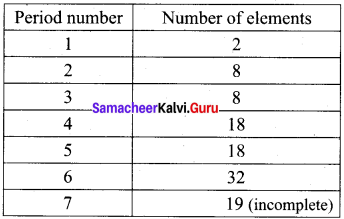The elements are shown in the above table along with its atomic numbe.
• The atomic number also indicates the number of electrons in the atoms of an element.
• This periodic table is important and useful because we can predict the properties of any element using periodic trend, even though that element may be unfamiliar to us.

Question 4.
Explain the merits of Moseley’s long form of periodic table.
Merits of Moseley’s long form of periodic table:

•  As this classification is based on atomic number, it relates the position of an element to its electronic configuration.
• The elements having similar electronic configuration fall in a group. They also have similar physical and chemical properties.
• The completion of each periõd is more logical. In a period as the atomic number increases, the energy shells are gradually filled up until an inert gas configuration is reached.
• The position of zero group is also justified in the table as group 18.
• The table completely separates metals and non-metals.
• The table separates two sub groups. lanthanides and actinides, dissimilar elements do not fall together.
• The greatest advantage of this periodic table is that this can be divided into four blocks namely s, p. d and f-block elements.
• This arrangement of elements is easier to remember, understand and reproduce.

Question 5.
Explain about the general characteristics of periods.
1. Number of electrons in outermost shell:
The number of electrons present in the outermost shell increases from 1 to 8 as we proceed in a period.

2. Number of shells:
As we move from left to right in a period the shells remains the same. The number of shells present in the elements corresponds to the period number. For example : all the elements of 2 period have on 2 shells (K, L)

3. Valency:
The valency of the elements increases from left to right in a period. With respect to hydrogen. the valency of period elements increases from 1 to 4 and then falls to one. With respect to oxygen, the valency increases from1 to 7.

4. Metallic character:
The metallic character of the elements decreases across a period.
For example: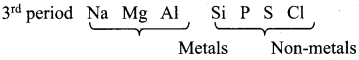Question 6.
Explain about the salient features of groups.
1. Number of electrons in outermost shell:
The number of electrons present in the outermost shells does not change on moving down in a group, i.e remains the same. Hence, the valency also remains same within a group.

2. Number of shells:
In going down a group the number of shells increases by one at each step and ultimately becomes equal to the period number to which the element belongs.

3. Valency:
The valencies of all the elements of the same group are the same. The valency of an element with respect to oxygen is same in a group.

4. Metallic character:
The metallic character of the elements increases in moving from top to bottom in a group.

Question 7.
Explain the classification of elements based on chemical behaviour and on physical properties.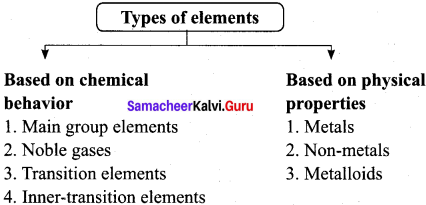Based of chemical behavior:

1. Main group elements:
All s-block and p-block elements excluding group elements are called representative elements.
2. Noble gases:
The 18th group elements are exclusively called noble gases. They have completely filled electronic configuration as ns2 np6. These elements are highly stable.
3. Transition elements:
The elements of d-block are called transition elements. These include elements of groups from 3 to 12 lying between s-block and p-block elements.
4. Inner transition elements.
The elements of f-block are called inner-transition elements. These consist of lanthanides and actinides, with 14 elements in each.

Based of physical properties:

1. Metals:
Metals comprise more than 78% of all known elements. They are usually solids at room temperature (except Hg, Ga and Cs). They have high melting and boiling points. They are good conductors of heat and electricity.
2. Non-metals:
Non-metals are usually solids or liquids or gases at room temperature with low melting and low boiling points (except boron and carbon). They are poor conductors of heat and electricity. Most of the non-metallic solids are brittle and are neither malleable nor ductile.
3.  Metalloids or Semi-metals:
Some elements in the periodic tables show properties that are characteristic of both metals and non-metals. They are called metalloids. Example : Silicon, germanium, arsenic, antimony and tellurium.

Question 8.
(b) What are the difficulties in determining atomic radius?
(a) Atomic radius is the distance between the center of its nucleus and the outermost shell
containing the electron.
(b) Difficulties in determining atomic radius

• The size of an atom is very small (∼ 1.2Å i.e 1.2 × 1010)
• The atom is not a rigid sphere; it is more like a spherical cotton ball rather than like a cricket ball.
•  It is not possible to isolate an atom and measure its radius.
• The size of an atom depends upon the type of atoms in its neighborhood and also the nature of bonding between them.

Question 9.
Prove that the atomic radii is a periodic property.
Atomic radius is the distance between the center of its nucleus and the outermost shell containing the electron.

Atomic radius is a periodic property.
1. Variation in periods:
The atomic radius decreases while going from left to right in a period. As we move from left to right in a period, the nuclear charge increases by one unit in each succeeding element. But the number of the shell remains same. Hence, the electrons are attracted strongly by the nucleus. Hence the atomic radius decreases along the period. In 2nd period rLi>rBe>rB>rC>rN>rO>rF

2. Variation in a group:
The atomic radius of elements increases with increase in atomic number as we move from top to bottom in a group. The attraction of the nucleus for the electrons decreases as shell number increases. Hence atomic radius increases along the group. In 1 group rLi < rNa < rK <rRb < rCs
Hence, atomic radii is a periodic property.

Question 10.
Explain about the factors that influence the ionization enthalpy. Factors influencing ionization enthalpy:
1. Size of the atom:
If the size of an atom is larger, the outermost electron shell from the nucleus is also larger and hence the outermost electrons experience lesser force of attraction. Hence it would be more easy to remove an electron from the outermost shell. Thus, ionization energy decreases with increasing atomic sizes.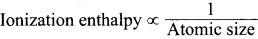2. Magnitude of nuclear charge:
As the nuclear charge increases, the force of attraction between the nucleus and valence electrons also increases. So, more energy is required to remove a valence electron. Hence I.E increases with increase in nuclear charge.
Ionization enthalpy α nuclear charge

3. Screening or shielding effect of the inner electrons:
The electrons of inner shells form a cloud of negative charge and this shields the outer electron from the nucleus. This screen reduces the coulombic attraction between the positive nucleus and the negative outer electrons. 1f screening effect increases, ionization energy decreases.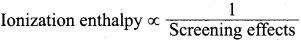4. Penetrating power of sub shells s, p, d and f:
The s-orbital penetrate more closely to the nucleus as compared to p-orbitais. Thus, electrons in s-orbitals are more tightly held by the nucleus than electrons in p-orbitais. Due to this, more energy is required to remove a electron from an s-orbital as compared to a p-orbital. For the same value of ‘n’, the penetration power decreases in a given shell in the order.
s > p > d > f.

5. Electronic configuration:
If the atoms of elements have either completely filled or exactly half filled electronic configuration, then the ionization energy increases.

Question 11.
Define ionization energy.
Prove that ionization energy is a periodic property.
(a) The energy required to remove the most loosely held electron from an isolated gaseous atom is called as ionization energy.
(b) (i) Variation in a period:
On moving across a period from left to right, the ionization enthalpy value increases. This is due to the following reasons:

• Increase of nuclear charge in a period
• Decrease of atomic size in a period

Because of these reasons, the valence electrons are held more tightly by the nucleus, thus ionization enthalpy increases. Hence, ionization energy is a periodic property.

(ii) Variation in a group:
As we move from top to bottom along a group, the ionization enthalpy decreases. This is due to the following reasons:

• A gradual increase in atomic size
• Increase of screening effect on the outermost electrons due to the increase of number of inner electrons.
Hence, ionization enthalpy is a periodic property.

Question 12.
Distinguish between electron affinity and electron negativity.
Electron affinity:

• It is the tendency of an isolated gaseous atom to gain an electron.
• Ills the property of an isolated atom.
• It does not change regularly in a period or a group.
• It is measured in electron volts/atom or kcal/mole or kJ/mole.

Electron negativity:

• It is the tendency of an atom in a molecule to attract the shared pair of electrons.
• It is the property of bonded atom.
• It changes regularly in a period or a group.
• It is a number and has no units.

Question 13.
What are the anomalous properties of second period elements?

1. In the Pt group, lithium differs in many aspects from its own family elements. Similarly, in the 2rd group, beryllium differs in many aspects from its own family.
2. For example. lithium forms compounds with more covalent character. But other alkali metals of this group form only ionic compounds.
3. Similarly, beryllium forms compounds with more covalent character while the other elements of this family form only ionic compounds.
4. Lithium and beryllium resemble more with the elements lying at their right hand side in the 3t1 period than with the other members of their own family.
5. These kinds of anomalies are also observed from 13th to 17th groups.
6. This sort of similarity is commonly referred to as diagonal relationship in the periodic properties.
7. The anomalous behaviors are attributed to the following factors:
• Smaller atomic size
• Higher ionization enthalpy
• High electronegativity

Activity 3.1

Covalent radii (in Å) for sonic elements of different groups and periods are listed below. Plot these values against atomic number. From the plot, explain the variation along a period and a group.
2nd group elements : Be (0.89), Mg (1.36), Ca (1.74), Sr (1.91) Ba( 1.98)
17th group elements : F (0.72), Cl (0.99), Br (l.14),I (1.33)
3nd Period elements : Na (1.57), Mg(1.36),AI (1.25), Si (1.17), P(1.10), S (1.04), Cl (0.99)
4thperiod elements : K (2.03), Ca (1.74), Sc (l.44), Ti(1.32), V (1.22), Cr (1.17), Mn (1.17), Fe( 1.17), CO (1.16), Ni (1.15), Cu (1.17), Zn(1.25), Ga(1.25), Ge(1.22), As(1.21), Se(1.14), Br( 1. 14)
Solution:
2nd group elements: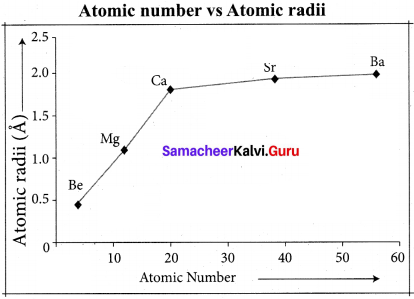17th group elements: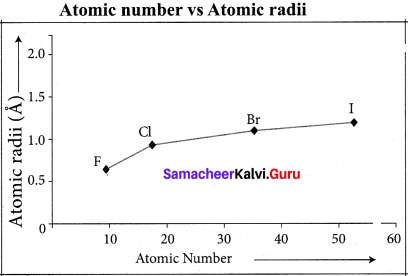As we move down the group, atomic radii increases with the increase in atomic number . As we move down the group, the number energy levels increases, as the number of electrons Periodic.

17th group elements: F (4.i), Cl (3.0), Br (2.8), I (2.5)
3rd Period elements : Na(0.9), Mg(l.2), Al (1.5), Si(l.8), P(2.1), S(2.5), Cl(3.0)
4th period elements : K(O.8), Ca (1.0), Sc (1.3), Ti (l.5), V(1.6), Cr(1.6), Mn(1.5), Fe(l.8), CO(1.9), Ni(1.9), Cu(1.9), Zn(1.6), Ga(1.6), Ge(1.8), As(2.0), Se(2.4), Br(2.8)
Solution:
2nd group elements: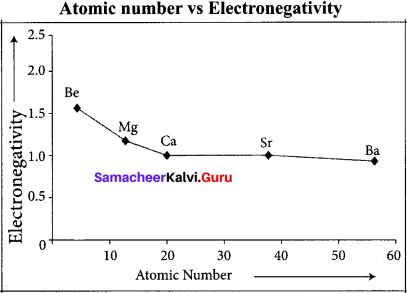17th group elements: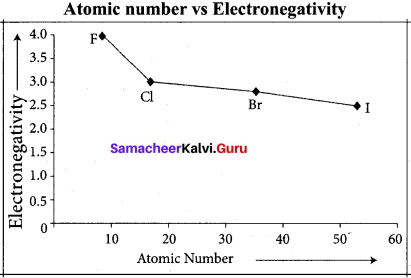As we go down the group, the electro negativity value decreases. Moving down the group, the electro negativity decreases due to the longer distance between the nucleus and the valence electron shell thereby decreasing the attraction making the atom have less attraction for electrons or protons.
3rd period: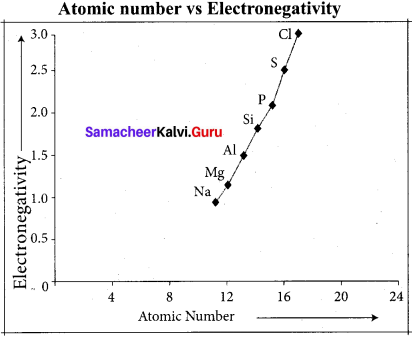4th period: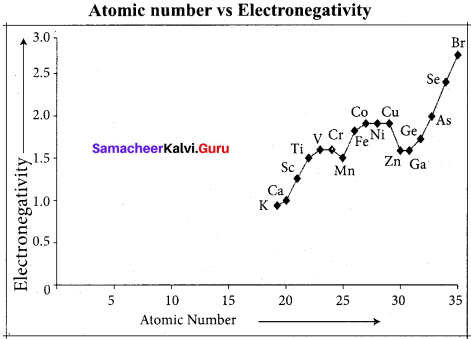The positively charged protons in the nucleus attract the negatively charged electrons. As the number of protons in the nucleus increases, the electro negativity or attraction will increase. Therefore electro negativity increases from left to right across the period. This occurs due to the greater charge on the nucleus, causing the electron bonding pairs to be very attracted to atoms placed further right on the periodic table.

## How to Prepare using Samacheer Kalvi 11th Chemistry Chapter 3 Periodic Classification of Elements Notes PDF?

Students must prepare for the upcoming exams from Samacheer Kalvi 11th Chemistry Chapter 3 Periodic Classification of Elements Notes PDF by following certain essential steps which are provided below.

• Use Samacheer Kalvi 11th Chemistry Chapter 3 Periodic Classification of Elements notes by paying attention to facts and ideas.
• Pay attention to the important topics
• Refer TN Board books as well as the books recommended.
• Correctly follow the notes to reduce the number of questions being answered in the exam incorrectly
• Highlight and explain the concepts in details.

## Frequently Asked Questions on Samacheer Kalvi 11th Chemistry Chapter 3 Periodic Classification of Elements Notes

#### How to use Samacheer Kalvi 11th Chemistry Chapter 3 Periodic Classification of Elements Notes for preparation??

Read TN Board thoroughly, make separate notes for points you forget, formulae, reactions, diagrams. Highlight important points in the book itself and make use of the space provided in the margin to jot down other important points on the same topic from different sources.

#### How to make notes for Samacheer Kalvi 11th Chemistry Chapter 3 Periodic Classification of Elements exam?

Read from hand-made notes prepared after understanding concepts, refrain from replicating from the textbook. Use highlighters for important points. Revise from these notes regularly and formulate your own tricks, shortcuts and mnemonics, mappings etc.
Share: